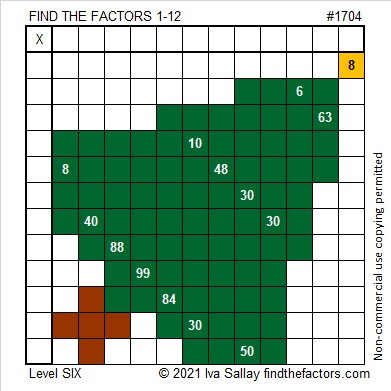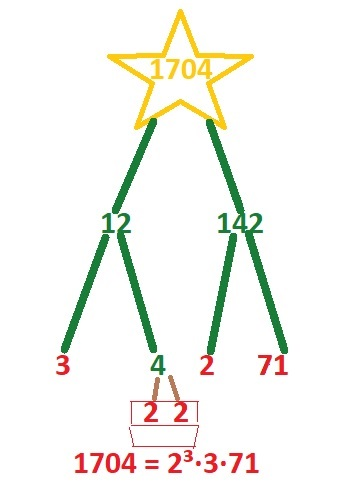# 1704 Christmas Factor Tree

Contents

### Today’s Puzzle:

If you know the factors of the clues in this Christmas tree, and you use logic, it is possible to write each number from 1 to 12 in both the first column and the top row to make a multiplication table. It’s a level six puzzle, so it won’t be easy, even for adults, but can YOU do it?### Factors of 1704:

If you were expecting to see a factor tree for the number 1704, here is one of several possibilities:• 1704 is a composite number.
• Prime factorization: 1704 = 2 × 2 × 2 × 3 × 71, which can be written 1704 = 2³ × 3 × 71.
• 1704 has at least one exponent greater than 1 in its prime factorization so √1704 can be simplified. Taking the factor pair from the factor pair table below with the largest square number factor, we get √1704 = (√4)(√426) = 2√426.
• The exponents in the prime factorization are 3,1 and 1. Adding one to each exponent and multiplying we get (3 + 1)(1 + 1) (1 + 1) = 4 × 2 × 2 = 16. Therefore 1704 has exactly 16 factors.
• The factors of 1704 are outlined with their factor pair partners in the graphic below.### More About the Number 1704:

1704 is the difference of two squares in FOUR different ways:
427² – 425² = 1704,
215² – 211² = 1704,
145² – 139² = 1704, and
77² – 65² = 1704.

Why was Six afraid of Seven? Because Seven ate Nine.
1704 is 789 in a different base:
1704₁₀ = 789₁₅ because 7(15²) + 8(15¹) + 9(15º) = 1704.

This site uses Akismet to reduce spam. Learn how your comment data is processed.